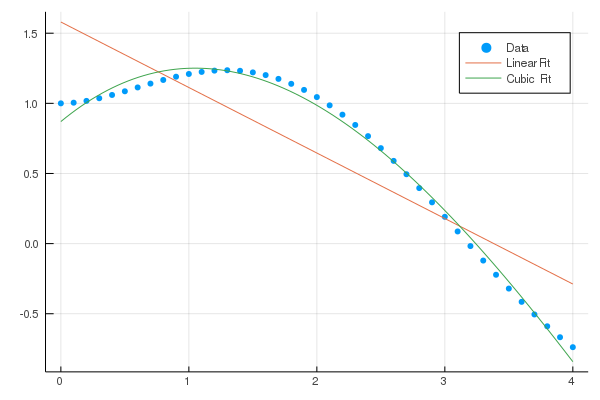95

7

38

30

# Polynomials.jl

Basic arithmetic, integration, differentiation, evaluation, and root finding over dense univariate polynomials.

## Installation

``````(v1.4) pkg> add Polynomials

julia> using Polynomials
``````

## Usage

#### Available Polynomials

``````* `Polynomial` - Standard basis polynomials, `a_0 + a_1⋅x + a_2⋅x^2 + ⋯ + a_n⋅xⁿ`,  `n ∈ ℕ`
``````
• `ImmutablePolynomial` - Standard basis polynomials backed by a tuple for faster evaluation of values
• `SparsePolynomial` - Standard basis polynomial backed by a dictionary to hold sparse high-degree polynomials
• `LaurentPolynomial` - Laurent polynomials, `a_m⋅x^m + ⋯ a_n⋅x^n` `m ≤ n`, `m,n ∈ ℤ` backed by an offset array
• `ChebyshevT` - Chebyshev polynomials of the first kind

#### Construction and Evaluation

Construct a polynomial from its coefficients, lowest order first.

``````julia> Polynomial([1,0,3,4])
Polynomial(1 + 3x^2 + 4x^3)
``````

An optional variable parameter can be added.

``````julia> Polynomial([1,2,3], :s)
Polynomial(1 + 2s + 3s^2)
``````

Construct a polynomial from its roots.

``````julia> fromroots([1,2,3]) # (x-1)*(x-2)*(x-3)
Polynomial(-6 + 11x - 6x^2 + x^3)
``````

Evaluate the polynomial `p` at `x`.

``````julia> p = Polynomial([1, 0, -1])
julia> p(0.1)
0.99
``````

#### Arithmetic

The usual arithmetic operators are overloaded to work on polynomials, and combinations of polynomials and scalars.

``````julia> p = Polynomial([1,2])
Polynomial(1 + 2x)

julia> q = Polynomial([1, 0, -1])
Polynomial(1 - x^2)

julia> 2p
Polynomial(2 + 4x)

julia> 2+p
Polynomial(3 + 2x)

julia> p - q
Poly(2x + x^2)

julia> p * q
Polynomial(1 + 2x - x^2 - 2x^3)

julia> q / 2
Polynomial(0.5 - 0.5x^2)

julia> q ÷ p  # `div`, also `rem` and `divrem`
Polynomial(0.25 - 0.5x)
``````

Note that operations involving polynomials with different variables will error.

``````julia> p = Polynomial([1, 2, 3], :x)
julia> q = Polynomial([1, 2, 3], :s)
julia> p + q
ERROR: Polynomials must have same variable.
``````

#### Integrals and Derivatives

Integrate the polynomial `p` term by term, optionally adding constant term `k`. The degree of the resulting polynomial is one higher than the degree of `p`.

``````julia> integrate(Polynomial([1, 0, -1]))
Polynomial(x - 0.3333333333333333x^3)

julia> integrate(Polynomial([1, 0, -1]), 2)
Polynomial(2.0 + x - 0.3333333333333333x^3)
``````

Differentiate the polynomial `p` term by term. The degree of the resulting polynomial is one lower than the degree of `p`.

``````julia> derivative(Polynomial([1, 3, -1]))
Polynomial(3 - 2x)
``````

#### Root-finding

Return the roots (zeros) of `p`, with multiplicity. The number of roots returned is equal to the degree of `p`. By design, this is not type-stable, the returned roots may be real or complex.

``````julia> roots(Polynomial([1, 0, -1]))
2-element Array{Float64,1}:
-1.0
1.0

julia> roots(Polynomial([1, 0, 1]))
2-element Array{Complex{Float64},1}:
0.0 - 1.0im
0.0 + 1.0im

julia> roots(Polynomial([0, 0, 1]))
2-element Array{Float64,1}:
0.0
0.0
``````

#### Fitting arbitrary data

Fit a polynomial (of degree `deg` or less) to `x` and `y` using a least-squares approximation.

``````julia> xs = 0:4; ys = @. exp(-xs) + sin(xs);

julia> fit(xs, ys) |> p -> round.(coeffs(p), digits=4) |> Polynomial
Polynomial(1.0 + 0.0593*x + 0.3959*x^2 - 0.2846*x^3 + 0.0387*x^4)

julia> fit(ChebyshevT, xs, ys, 2) |> p -> round.(coeffs(p), digits=4) |> ChebyshevT
ChebyshevT(0.5413⋅T_0(x) - 0.8991⋅T_1(x) - 0.4238⋅T_2(x))
``````

Visual example:#### Other methods

Polynomial objects also have other methods:

• 0-based indexing is used to extract the coefficients of `[a0, a1, a2, ...]`, coefficients may be changed using indexing notation.

• `coeffs`: returns the entire coefficient vector

• `degree`: returns the polynomial degree, `length` is number of stored coefficients

• `variable`: returns the polynomial symbol as polynomial in the underlying type

• `norm`: find the `p`-norm of a polynomial

• `conj`: finds the conjugate of a polynomial over a complex field

• `truncate`: set to 0 all small terms in a polynomial;

• `chop` chops off any small leading values that may arise due to floating point operations.

• `gcd`: greatest common divisor of two polynomials.

• `Pade`: Return the Pade approximant of order `m/n` for a polynomial as a `Pade` object.

## Legacy code

As of v0.7, the internals of this package were greatly generalized and new types and method names were introduced. For compatability purposes, legacy code can be run after issuing `using Polynomials.PolyCompat`.

## Contributing

If you are interested in contributing, feel free to open an issue or pull request to get started.

02/24/2014

3 days ago

418 commits